# To Prove or Not to Prove

##### Age 14 to 18

Published 2001 Revised 2008

## Introduction

There is a legendary story of the sage who posed the question: 'A normal elephant has four legs; if an elephant's trunk is called a leg, how many legs does it have?' He asked a mathematician, who continued to stare at a pile of paper on which he was scribbling as he muttered: 'four and one make five'. Next to him a philosopher mused enigmatically and puffed for a few moments on his pipe before observing: 'The fact that it is called a leg, doesn't change the fact that it is not a leg, so the answer is four '. 'Excuse me,' said a passing zoologist, 'if a trunk is classified as a leg, clearly this will also apply to the tail, so it has six legs, and it's an insect'. A logician joined the conversation: 'A normal elephant has four legs, but you did not actually say that this elephant is normal, so there is insufficient evidence...'

Continuing to seek enlightenment, the sage in his wisdom passed the query on to a statistician who returned the following day asserting 'the mean is 0.33'. 'Might I ask how you came by this information?' queried the sage, concealing his innermost thoughts behind an inscrutible smile. 'The best way to solve such a question is to obtain empirical information,' replied the statistician, 'so I went to the local zoo and got the answer from the horse's mouth, so to speak. Two elephants refused to respond and the third blew his own trumpet just once.'

Still bemused the sage went along to the local school which was deeply embroiled in GCSE investigations and once again stated his problem. 'That's a very interesting question,' said the teacher.

The moral of this story is that, as Humpty Dumpty once said, 'when I use a word, it means just what I want it to mean, and nothing else'. The term 'proof' is just such a word. In different contexts it means very different things. To a judge and jury it means something established by evidence 'beyond a reasonable doubt'. To a statistician it means something occuring with a probability calculated from assumptions about the likelihood of certain events happening randomly. To a scientist it means something that can be tested -- the proof that water boils at 100o is to carry out an experiment. A mathematician wants more -- simply predicting and testing is not enough -- for there may be hidden assumptions (that the water boiling is always carried out at normal atmospheric pressure and not, say, on the top of Mount Everest).

## Problem Solving and Convincing Arguments

When a problem is encountered, the question of providing a convincing argument to explain the solution often arises. The book 'Thinking Mathematically' by John Mason, Leone Burton and Kay Stacey has a large number of problem-solving situations. One is the problem 'into how many squares can you cut a square?'

Faced with such a question, you might begin by thinking 'as many as you like', or 'infinity'. Then you may begin to realize that a square could be cut into 4, 9 or 16 by dividing it into pieces of equal size. If you play about with possible ways of cutting a square into smaller squares, you may suddenly see that any square can be be cut into four smaller squares, including the smaller squares themselves. Aha! A square could be cut into four quarters and one quarter cut into four again, losing the quarter as a counted square but gaining four smaller ones - so a square can be cut into seven squares.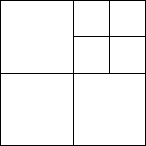Figure 1: cutting a square into seven squares

Cutting any of the squares in this picture into four squares gives three extra squares. Thus it is possible to cut a square into 7 squares, 10 squares, 13 squares, 16, 19, 22, ... and so on.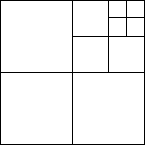Figure 2: cutting a square into ten squares

It is easy to see that IF a square can be cut into n squares THEN it can be cut into n+3 squares. It is this general result which is the key to the solution of the problem. For instance, if I could cut a square into six smaller squares, then I could do 6+3=9, 9+3=12, and so on, to get the sequence 6, 9, 12, 15, ... If I could cut a square into five smaller squares, then I could get the sequence 5, 8, 11, 14, ..., and so on. But can I ?

One attack suggested by students is to look at a picture like figure 3, and to propose that, by rubbing out a number of lines in a three by three subdivision it is possible to "glue four squares into a single square". This gives one big square and five smaller squares, making six squares in all.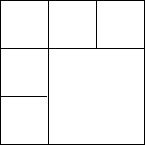Figure 3: cutting a square into six squares (one big, five small)

This can then be built on by subdividing any of these squares into four smaller, to cut a square into 6+3=9, 9+3=12, ... to get the sequence of possibilities: 6, 9, 12, 15, ...

If you could cut a square into five squares, it would be possible to get the sequence of possibilities 5, 8, 11, 14, ... Then it would be possible to do all possible numbers 4 and above, using the combination of the three sequences

4, 7, 10, 13, ...
5, 8, 11, 14, ...
6, 9, 12, 15, ...

But can you cut a square into five smaller squares? One student, Paul, suggested to me that if you can do n squares you can do n-3 by joining a block of four squares together as in Figure 3. Is Paul's suggestion correct? It is certainly true for n=9, as Figure 3 shows, but is it true for all whole numbers n?

There is a well-known story of the experimental physicist who claimed to prove that 60 is divisible by every other number. He came to this conclusion by considering a sequence of cases to establish the pattern: 1,2,3,4,5,6 and then moved on to a few others at random to test out the theory : 10,12, 20, 30, and concluded that his result was experimentally verified. He was surpassed in this endeavour by an engineer who noticed that all odd numbers seemed to be prime... One - well that's an oddity, but we'll include it in - three, five, seven, good, we're getting somewhere - nine? Oh, nine... Let's leave that a moment - eleven, thirteen - fine. The exceptional case of nine must have been an experimental error.

This story, which I claim bears no relationship to any known physicist or engineer, living or dead, does illustrate the important difference between proof by looking at a number of cases and proper mathematical proof. It is not enough to consider just a number of cases, for all of them may have some hidden common assumption. For instance, we might conclude from a number of experiments that water always boils at 100o C because we never have the experience of trying to boil water on the top of Mount Everest. Scientific proof depends on the predictability of experiments: that we conjecture that when we carry out an experiment it will have a predicted outcome. Such proof is not appropriate in mathematics where we must provide a logical argument that the conclusion follows from explicitly stated assumptions.

To help the student focus on the various stages of putting up a convincing argument, 'Thinking Mathematically' suggests three stages:

1. Convince yourself.
2. Convince a friend.
3. Convince an enemy.

The idea is first to get a good idea how and why the result works, sufficient to believe its truth. Convincing oneself is, regrettably, all too easy. So pleased is the average mortal when the 'Aha!' strikes that, even if shouting 'Eureka' and running down the street in a bath towel is de rigeur, it is very difficult to believe that the blinding stroke of insight might be wrong. So the next stage is to convince a friend - another student, perhaps - which has the advantage that, to explain something to someone else at least makes one sort out the ideas into some kind of coherent argument. The final stage in preparing a convincing argument, according to 'Thinking Mathematically' is to convince an enemy - a mythical arbiter of good logic who subjects every stage of an argument with a fine toothcomb to seek out weak links.

A student might very well convince himself of the truth of the argument "IF I can cut a square into n smaller squares, THEN I can cut the square into n- 3 squares". He might even convince a friend by showing pictures such as figure 3. But an enemy might put up figure 4, where a square is cut into eight smaller squares (seven the same size, plus one bigger one). Here there is no set of four smaller subsquares in a group which can be amalgamated into one larger square to reduce the eight sub-squares to five sub-squares.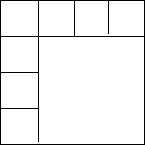Figure 4: cutting a square into eight squares, but not into five

Does this blow, which demolishes Paul's theory, show that you cannot cut a square into five smaller squares? No it does not. It suggests that Paul's method does not work, but perhaps some other method will ...

The problem which I leave you to formulate precisely and prove has two parts:

(a) Find all numbers n such that a square can be cut into n smaller sub-squares and prove that this is actually possible for every such number n.

(b) For all the numbers n not included in part (a), prove that it is not possible to cut a square into such a number of smaller squares.

You should certainly have a go at this before moving to the next section.

## Making Precise Statements

Proof requires a careful statement of assumptions and a precise argument showing how a clearly stated result is deduced. It is surprising how often we miss the fact that a statement has implicit, unspoken assumptions. Look at the square problem. Into how many squares can I cut a square? For what numbers is this not possible?

If a square is cut into more than one square, there will be a corner of a smaller square in each corner of the original square. Thus, if there is more than one square, there must be at least four . There cannot be two or three. Perhaps you might like to try to extend this argument to cover other cases which you suspect cannot be done (if there are any...).

I have given this problem to hundreds of undergraduates over the years and we have all eventually agreed on which values of n cannot be done. It has become quite a party piece which I have also tried out with many sixthformers.

It was ten years after I first met the problem that a perceptive fourteen year old girl in a problem-solving session came up with an original thought. She suggested that the problem had not explicitly stated that the paper could not be cut and then glued together again in a different way. Her solution for n=2 is given in figure 5.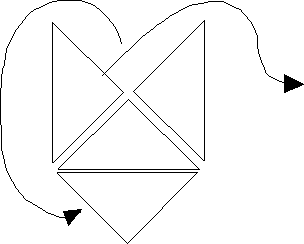Figure 5: sticking together bits to cut a square into two squares

This illustrates the fact that we must be extremely careful about how we phrase our assumptions. Before Figure 5 the (unspoken) assumption had been that we must make single straight line cuts to form whole subsquares and we are not allowed to cut into smaller parts (say triangles) and to stick them together again. The original problem is better specified by saying:

Square problem version 2 : A square is cut into n smaller squares by making single straight line cuts, without joining together cut parts into larger wholes. What are the possible values of n?

The exceptional cases found earlier would still be exceptions to this better phrased problem. Figure 5 would now fail to be a counter example to this because it breaks the rule about not sticking together cut parts into larger wholes.

However, Figure 5 does suggest a different problem:

Square problem version 3 : Into how many subsquares n is it possible to cut a square, if it is allowed to join cut parts into large wholes?

The answer to problem version 3 is likely to be different from that to version 2. You should see if any or all of the 'impossible' numbers from version 2 now become 'possible'. For instance, in Figure 4 we can clearly take any four of the smaller squares and move them together to glue them into one medium size square. Thus, if we allow sticking together we can re-form the square in Figure 4 into five squares of different sizes: one large, one medium, and three little ones. With a little ingenuity perhaps you can solve the case n=3 for version 3 of the problem. Perhaps now you can specify the solutions of both problems. They will be different. This shows that precision in making mathematical statements is all important.

Three men were going by train to a conference in a distant region of the United Kingdom. The engineer looked out of the window and said, 'Look, all the sheep in Scotland are black'. The theoretical physicist thought for a moment and said, 'No, there exists a field in Scotland in which all the sheep are black'. There was silence from the logical mathematician who mused for some time in the corner of the compartment before declaring 'No, there exists a field in Scotland in which all the sheep are at least half black...'

### Making appropriate deductions

Once we have got precise statements of the assumptions (P) underlying a theorem and what it is we are trying to prove (Q), then a mathematical proof of the theorem is in the form "IF P is true THEN Q is true". In everyday language the conventions are sometimes different. "If your father comes home before six o'clock then you can have some chocolate before dinner-time". Here the assumption P is "father comes home before six o'clock" and the deduction Q is "you can have chocolate before dinner-time". Presumably father brings the chocolate and if he arrives sufficiently early you can have some without spoiling your appetite. But also contained in the statement that IF father does not come home before six o'clock, THEN you will NOT have chocolate before dinner-time. There is often an implication in everyday language that IF P happens THEN Q will follow, but IF P FAILS THEN Q FAILS ALSO.

In mathematics such an assumption is not made. Here a proof in the form IF P THEN Q simply requires that if P is true, then Q must be true also. If P is false, then no implication as to the truth or falsehood of Q is necessary.

Consider the example:

If x > 6 then x > 3 .

In mathematics this is considered a true statement. If x is a number bigger than 6 then it must also be bigger than 3. However, consider this as separate statements, where P is " x > 6 " and Q is "x > 3 ". What happens for various values of x? If x=7 then P is true and Q is also true. In fact, when x is a number bigger than 6 then P is true and it will follow also that Q is true.

But if x=5 then P is false but Q is true, and if x=3 then P is false and Q is false. Thus when P is false, Q can be true or false. We simply have no interest what happens in this case.

## Common Errors in Proof

Students often make quite serious errors in proof on examination papers. As a Senior Examiner in Mechanics for many years I regularly had to mark questions which said something like this:

A particle mass M rests on a rough plane with coefficient of friction $\mu$, inclined to the horizontal at an angle $\alpha$. Show that if the particle slides down the plane then $\tan\alpha> \mu$.
What students often do is to assume $\tan\alpha> \mu$ and deduce that the particle slides. They have been asked to prove IF P THEN Q where P is "the particle slides" and Q is "$\tan\alpha> \mu$". They often prove IF Q THEN P. In this case it happens that the two things are equivalent. P happens if and only if Q happens. But the question only asks for the implication from P to Q and the students only prove the implication from Q to P.

You might feel that this is a trivial matter. But logically it is totally erroneous. In mathematics it often happens that IF P THEN Q is true but IF Q THEN P is false. For instance, it is true that IF x > 6 THEN x > 3 , but the other way round: IF x > 3 THEN x > 6 is clearly false. Thus it is important to distinguish between the two. The statement IF Q THEN P is called the converse of the statement IF P THEN Q. It is important to distinguish between the proof of a statement and the proof of its converse. One may be true and the other may be false. Another example occurred with the case of "into how many squares can I cut a square" (version 2). It is true to say that if a square can be cut into n pieces then it can be cut into n+3 pieces. The converse, that if it can be cut into n+3 pieces it can be cut into n pieces is false, as can be seen from the case n=3,5.

There is one case in A-level that (almost) everyone gets wrong. It is to do with the constant of integration: that if

$\int f(x) dx = F(x)$,

then any other integral is of the form

$F(x)+c$

where $c$ is a constant. This is usually deduced from the fact that the derivative of a constant $c$ is zero. Hence the derivative of

$F(x)+c$

is the same as the derivative of

$F(x)$.

However, the deduction is false. Let $P$ be the statement that

$G(x)=F(x)+c$

and $Q$ be the statement

$G\prime(x)=F\prime(x)$.

Then, because the derivative of a constant is zero we can deduce that IF $P$ is true THEN $Q$ is true. What we cannot do is to deduce the converse: IF $Q$ is true THEN $P$ is true.

It is actually possible to have $Q$ true and $P$ false. As an example, let

$G(x)=1/x$

and let
\begin{eqnarray}F(x) =& 1/x+1 (x < 0) \\ & 1/x+2 (x > 0) \end{eqnarray}

then both $G(x)$ and $F(x)$have derivative $-1/x^2$. The fact that there is a different constant added to $F(x)$ for x < 0 and x > 0 does not affect the derivative because these two parts of the domain are totally separate. (In forming the limit of $(F(x+h)-F(x))/h$, as $h$ tends to zero, when $h$ is sufficiently small, both $F(x)$ and $F(x+h)$ will have the same added constant.)

Oh, you may say, that's cheating, we don't normally meet functions like that in the calculus... No we don't. Nor do we normally have the personal experience of boiling water on the top of Mount Everest, which would prove that water doesn't always boil at 100o C.

To be sure that the mathematics will always work it is necessary to state precisely the assumptions and to take great care over the deductions. This proves to be rather hard. Indeed it tends to be the province of university pure mathematics rather than A-level.

You may find the accent on precise proof in mathematics rather esoteric. Other scientists are known to make such jibes at mathematicians. Indeed they say you can tell whether someone is an engineer, physicist or mathematician by setting fire to his wastepaper basket. The engineer will make a cursory calculation and swamp the basket with enough water to put out the fire and more. The physicist will sit down, calculate exactly how much water is needed and pour the exact quantity on the fire. The mathematician? The mathematician will sit down and calculate exactly how much water is needed.

Thus the mathematician stands accused of developing a precise theory that is devoid of application. This could not be further from the truth. In our universities computer scientists are growing increasingly worried that students no longer seem to understand the finer points of proof. This is especially true since the demise of Euclidean geometry which was largely concerned with the ritual of deducing one statement about a geometrical figure from given assumptions. It may have serious consequences. As we use increasingly more sophisticated software to run our lives we need computer scientists and programmers who can write provably correct software that does not contain horrendous bugs - unlike the kind of software that caused the stockmarket crash because it was designed to sell under certain conditions which occurred late one Friday and caused the computers to attempted to outdo each other as the selling fed back into the system causing even more selling and then eventual collapse of the market.

It is therefore even more important in today's technological climate to pay attention to the niceties of well-formulated statements and logical deduction. "To prove or not to prove" is a question that can have only one answer, for proof is an essential component of technological order in the future.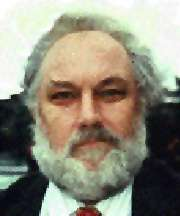Professor David Tall

Professor in Mathematical Thinking at the University of Warwick (1992-present).

Educated at Victoria School (1945-1952), The Grammar School, Wellingborough (1952-1960) and Wadham College Oxford (1960-1966) where I obtained first class honours in Mathematics, The Junior Mathematics Prize, (1963) and DPhil in Mathematics (1967). From 1966 to 1969 I was a lecturer in Mathematics at Sussex University. Since 1969, I have been on the staff of the University of Warwick, as a lecturer in mathematics with special interests in education in the Mathematics Institute (1969-1980), then within what has become the Institute of Education, being awarded a personal chair in 1992.

[This article was originally published in Mathematics Review in January 1991. NRICH has the permission of the editors and authors to reprint material from it as the Mathematics Review magazine closed down after 17 issues. Ed.]